# annotation

## 语法

``annotation(lineType,x,y)``
``annotation(lineType)``
``annotation(shapeType,dim)``
``annotation(shapeType)``
``annotation(___,Name,Value)``
``annotation(container,___)``
``an = annotation(___)``

## 说明

``annotation(lineType,x,y)` 创建一个在当前图窗中的两个点之间延伸的线条或箭头注释。将 `lineType` 指定为 `'line'`、`'arrow'`、`'doublearrow'` 或 `'textarrow'`。将 `x` 和 `y` 分别指定为 `[x_begin x_end]` 和 `[y_begin y_end]` 形式的二元素向量。`
``annotation(lineType)` 在点 `(0.3,0.3)` 和 `(0.4,0.4)` 之间的默认位置创建注释。`

``annotation(shapeType,dim)` 在当前图窗中创建具有特定大小和位置的矩形、椭圆或文本框注释。将 `shapeType` 指定为 `'rectangle'`、`'ellipse'` 或 `'textbox'`。指定 `dim` 作为 `[x y w h]` 形式的四元素向量。`x` 和 `y` 元素确定位置，`w` 和 `h` 元素确定大小。`
``annotation(shapeType)` 会在默认位置创建注释，以使左下角位于 `(0.3,0.3)` 且宽度和高度均为 `0.1`。`

``annotation(___,Name,Value)` 用于创建注释并将属性指定为名称-值对组参数。不同类型的注释支持不同属性。您可以使用上述语法中的任意输入参数组合指定属性。`
``annotation(container,___)` 在由 `container` 指定的图窗、uipanel 或 uitab 中创建注释，而不是在当前图窗中创建。`

``an = annotation(___)` 返回注释对象。返回的对象的类型取决于第一个输入参数。创建对象之后，可使用 `an` 修改其属性。可以使用上述任意语法指定输出参数。 `

## 示例

```figure plot(1:10) x = [0.3 0.5]; y = [0.6 0.5]; annotation('textarrow',x,y,'String','y = x ')```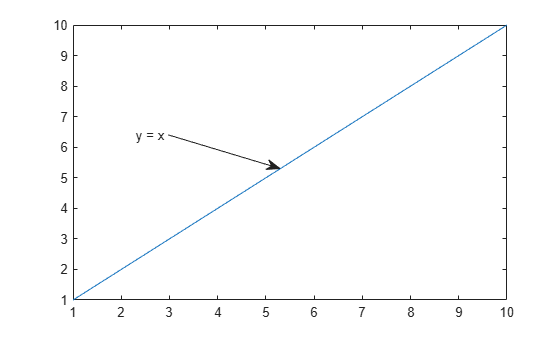```figure plot(1:10) dim = [.2 .5 .3 .3]; str = 'Straight Line Plot from 1 to 10'; annotation('textbox',dim,'String',str,'FitBoxToText','on');```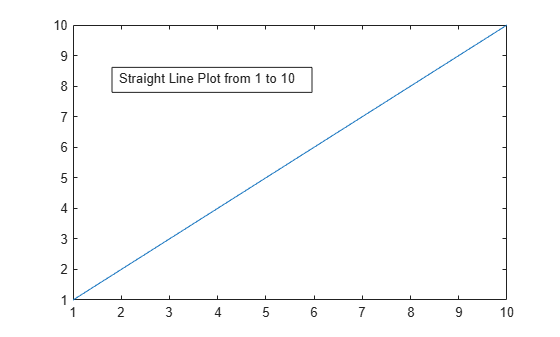```figure plot(1:10) dim = [.2 .5 .3 .3]; str = 'Straight Line Plot from 1 to 10'; annotation('textbox',dim,'String',str)```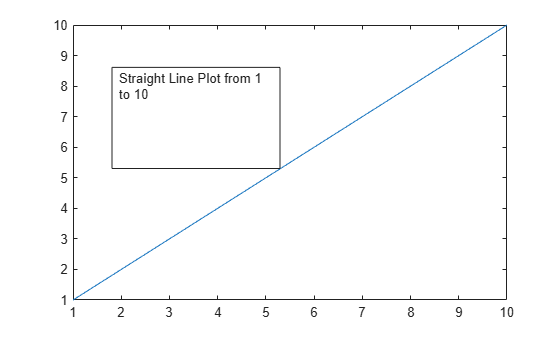```figure plot(1:10) dim = [0.2 0.5 0.3 0.3]; str = {'Straight Line Plot','from 1 to 10'}; annotation('textbox',dim,'String',str,'FitBoxToText','on');```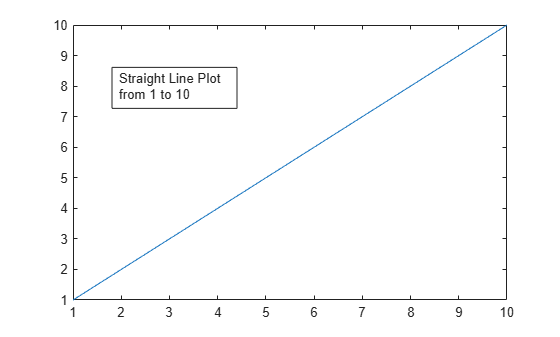```figure data = [2 4 6 7 8 7 5 2]; stem(data) dim = [.3 .68 .2 .2]; annotation('rectangle',dim,'Color','red')```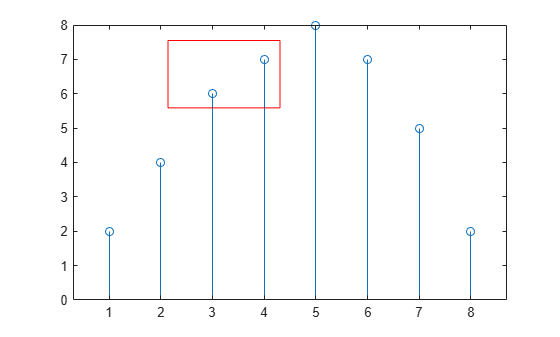```dim2 = [.74 .56 .1 .1]; annotation('rectangle',dim2,'FaceColor','blue','FaceAlpha',.2)```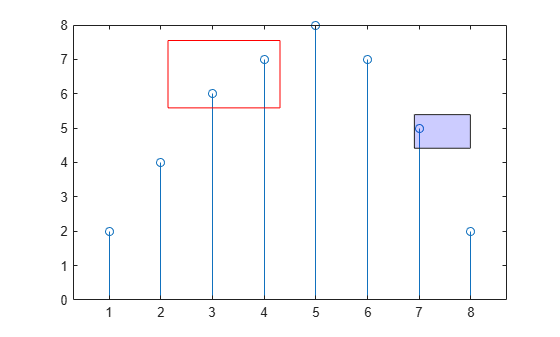```figure x = linspace(-4,4); y = x.^3 - 12*x; plot(x,y) dim = [.2 .74 .25 .15]; annotation('ellipse',dim)```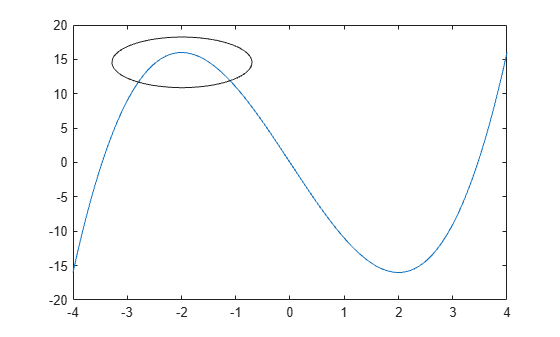`annotation('rectangle',dim,'Color','red')`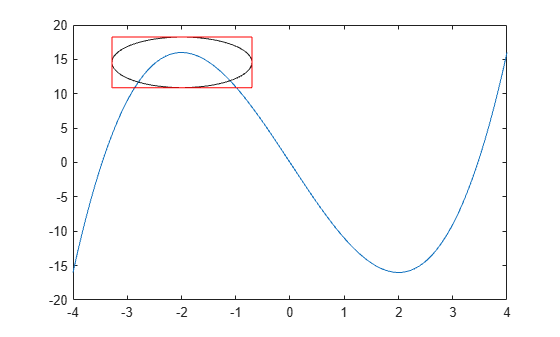```figure plot(1:10) xl = [.3 .3]; yl = [.3 .4]; annotation('line',xl,yl) xa = [.3 .4]; ya = [.4 .4]; annotation('arrow',xa,ya)```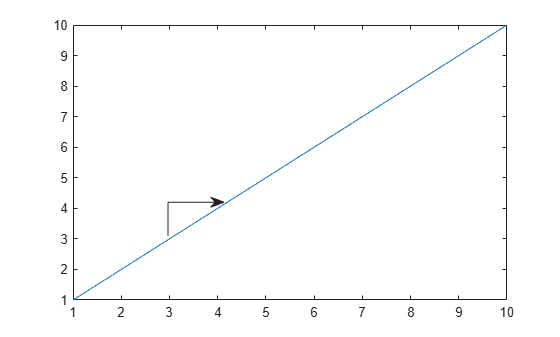```figure plot(1:10) x = [0.3,0.5]; y = [0.6,0.5]; a = annotation('textarrow',x,y,'String','y = x ');``````a.Color = 'red'; a.FontSize = 14;```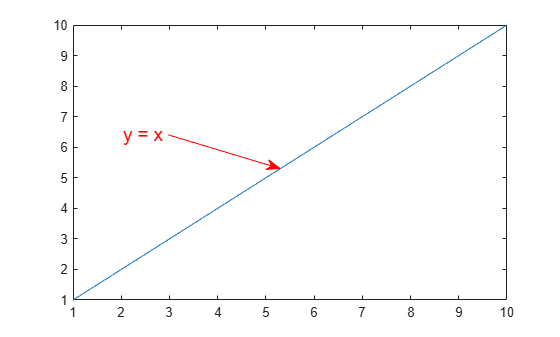## 输入参数

`'line'`注释线条`annotation('line',[.1 .2],[.1 .2])`
`'arrow'`注释箭头`annotation('arrow',[.1 .2],[.1 .2])`
`'doublearrow'`注释双箭头`annotation('doublearrow',[.1 .2],[.1 .2])`
`'textarrow'`

`annotation('textarrow',[.1 .2],[.1 .2],'String','my text')`

`'rectangle'`注释矩形`annotation('rectangle',[.2 .3 .4 .5])`
`'ellipse'`注释椭圆`annotation('ellipse',[.2 .3 .4 .5])`
`'textbox'`

`annotation('textbox',[.2 .3 .4 .5],'String','my text','FitBoxToText','on')`

```f = figure; annotation(f,'line',[.1 .2],[.2 .3])```

## 输出参数

`'line'`线条Line 属性
`'arrow'`箭头Arrow 属性
`'doublearrow'`双箭头DoubleEndArrow 属性
`'textarrow'`文本箭头TextArrow 属性
`'textbox'`文本框TextBox 属性
`'rectangle'`矩形Rectangle 属性
`'ellipse'`椭圆Ellipse 属性